Next: Spatial Curvature Up: Some Consequences Previous: The Big Bang

### The Cosmological Constant

The simplified big bang model just described is inaccurate for the very early history of the universe, when the pressure of radiation was important. Moreover, recent observations seem to indicate that it is seriously inaccurate even in the present epoch. First of all, it seems that much of the energy density is not accounted for by known forms of matter. Still more shocking, it seems that the expansion of the universe may be accelerating rather than slowing down! One possibility is that the energy density and pressure are nonzero even for the vacuum. For the vacuum to not pick out a preferred notion of rest', its stress-energy tensor must be proportional to the metric. In local inertial coordinates this means that the stress-energy tensor of the vacuum must be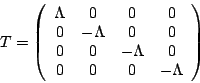where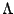is called the cosmological constant'. This amounts to giving empty space an energy density equal toand pressure equal to, so thatfor the vacuum is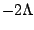. Here pressure effects dominate because there are more dimensions of space than of time! If we add this cosmological constant term to equation (3), we get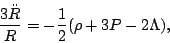(4)

whereandare the energy density and pressure due to matter. If we treat matter as we did before, this gives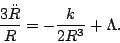Thus, once the universe expands sufficiently, the cosmological constant becomes more important than the energy density of matter in determining the fate of the universe. If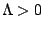, a roughly exponential expansion will then ensue. This seems to be happening in our universe now.

Next: Spatial Curvature Up: Some Consequences Previous: The Big Bang

© 2006 John Baez and Emory Bunn